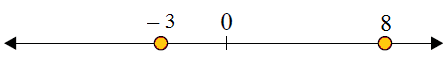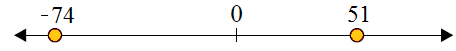# Using a number line to compare integers

Compare the integers using number line. Use the symbols: <, >, and =.

8 __ −3

### Solution

Step 1:

On the number line 8 lies to the right of −3. So 8 > −3

Step 2:

On number lineCompare the integers using number line. Use the symbols: <, >, and =.

51 __− 74

### Solution

Step 1:

On the number line −74 lies to the left of 51. So −74 < 51

Step 2:

On number line